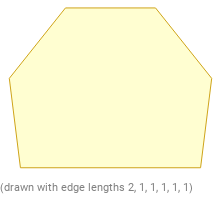Search IntMath
Close

450+ Math Lessons written by Math Professors and Teachers

5 Million+ Students Helped Each Year

1200+ Articles Written by Math Educators and Enthusiasts

Simplifying and Teaching Math for Over 23 Years

# Hexagons in GeometryHexagons are one of the most commonly used polygon shapes in mathematics and geometry. A polygon is a two-dimensional shape, composed of straight lines, with three or more sides. Hexagons are a type of polygon that has six sides and six angles. All of the hexagon's angles are equal, and each side has the same length.

## Types of Hexagonal Shapes

There are two types of hexagons: regular and irregular. Regular Hexagons are made up of six equal sides and angles. The length of each side is the same and all of the angles are equal. Irregular hexagons are made up of six sides, but the length of each side and the angles are not necessarily the same.

## Calculating the Perimeter of a Hexagon

The perimeter of a hexagon is the total length of all of its sides. To calculate the perimeter of a regular hexagon, you need to know the length of one of its sides. Then, you can multiply that length by 6, because it has 6 sides. For example, if one side of a regular hexagon is 4 inches, then the perimeter of the hexagon will be 24 inches (4 * 6 = 24).

## Calculating the Area of a Hexagon

The area of a hexagon is the amount of space inside the shape. To calculate the area of a regular hexagon, you need to know the length of one of its sides. First, you will need to calculate the hexagon's apothem, which is the distance from the center of the hexagon to one of its sides. To determine the apothem, divide the length of one side by 2 and then take the square root of that number. Then, you can multiply the length of one side by the apothem and then multiply that number by 6 (because it has 6 sides). For example, if one side of a regular hexagon is 4 inches, then the area of the hexagon will be 83.2 square inches (4 * sqrt (4/2) * 6 = 83.2).

## Practice Problems

1. Calculate the perimeter of a regular hexagon with a side length of 6 inches.
Answer: 36 inches (6 * 6 = 36)
2. Calculate the area of a regular hexagon with a side length of 8 inches.
Answer: 176.7 square inches (8 * sqrt (8/2) * 6 = 176.7)
3. Calculate the perimeter of an irregular hexagon with side lengths of 4, 7, 8, 3, 4, and 9 inches.
Answer: 35 inches (4 + 7 + 8 + 3 + 4 + 9 = 35)
4. Calculate the area of an irregular hexagon with side lengths of 5, 6, 3, 4, 7, and 9 inches.
Answer: 89.1 square inches (5 * sqrt (5/2) + 6 * sqrt (6/2) + 3 * sqrt (3/2) + 4 * sqrt (4/2) + 7 * sqrt (7/2) + 9 * sqrt (9/2) = 89.1)
5. Calculate the perimeter of a regular hexagon with a side length of 12 inches.
Answer: 72 inches (12 * 6 = 72)
6. Calculate the area of a regular hexagon with a side length of 5 inches.
Answer: 64.8 square inches (5 * sqrt (5/2) * 6 = 64.8)
7. Calculate the perimeter of an irregular hexagon with side lengths of 3, 4, 6, 5, 8, and 7 inches.
Answer: 33 inches (3 + 4 + 6 + 5 + 8 + 7 = 33)
8. Calculate the area of an irregular hexagon with side lengths of 4, 6, 3, 5, 7, and 9 inches.
Answer: 103.9 square inches (4 * sqrt (4/2) + 6 * sqrt (6/2) + 3 * sqrt (3/2) + 5 * sqrt (5/2) + 7 * sqrt (7/2) + 9 * sqrt (9/2) = 103.9)

## Summary

Hexagons are a type of polygon shape with six sides and six angles. There are two types of hexagons: regular and irregular. To calculate the perimeter of a hexagon, you need to know the length of one of its sides and then multiply that number by 6. To calculate the area of a hexagon, you need to calculate the hexagon's apothem and then multiply the length of one side by the apothem and then by 6. In this article, we discussed the basics of hexagons, how to calculate their perimeters and areas, and provided some practice problems and answers.

## FAQ

### What is a hexagon explained for kids?

A hexagon is a shape with six sides and six angles. Each side is a straight line, and each angle is the same size. Hexagons are often found in nature, such as in the shape of honeycombs, and can also be found in man-made objects such as tiles.

### What are the 5 properties of a hexagon?

The five properties of a hexagon are: It has six sides, six angles, all sides are equal in length, all angles are equal in size, and it has an internal angle of 120 degrees.

### What is a hexagon shape called?

The hexagon shape is called a hexagon, or sometimes a hex or hexahedron.

### What is a hexagon and its properties?

A hexagon is a shape with six sides and six angles. All sides are equal in length and all angles are equal in size. The internal angle of a hexagon is 120 degrees.

## Problem SolverThis tool combines the power of mathematical computation engine that excels at solving mathematical formulas with the power of GPT large language models to parse and generate natural language. This creates math problem solver thats more accurate than ChatGPT, more flexible than a calculator, and faster answers than a human tutor. Learn More.